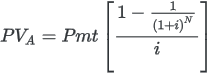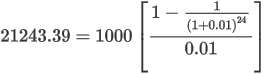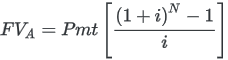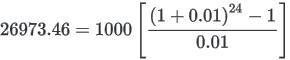# PMT( ) function

Returns the amount of the periodic payment (principal + interest) required to pay off a loan.

## Syntax

`PMT(rate, periods, amount <,type>)`

## Parameters

Name Type Description
rate numeric

The interest rate per period.

periods numeric

The total number of payment periods.

amount numeric

The principal amount of the loan.

type

optional

numeric

The timing of payments:

• 0 – payment at the end of a period
• 1 – payment at the beginning of a period

If omitted, the default value of 0 is used.

Note

You must use consistent time periods when specifying rate and periods to ensure that you are specifying interest rate per period.

For example:

• for monthly payments on a two-year loan or investment with interest of 5% per annum, specify 0.05/12 for rate and 2 * 12 for periods
• for annual payments on the same loan or investment, specify 0.05 for rate and 2 for periods

Numeric.

## Examples

### Basic examples

Returns 1856.82, the monthly payment (principal + interest) required to pay off a twenty-five year, \$275,000 loan at 6.5% per annum, with payments due at the end of the month:

`PMT(0.065/12, 12*25, 275000, 0)`

Returns 1846.82, the monthly payment (principal + interest) required to pay off the same loan, with payments due at the beginning of the month:

`PMT(0.065/12, 12*25, 275000, 1)`

### Annuity calculations

Annuity calculations involve four variables:

• present value, or future value \$21,243.39 and \$ 26,973.46 in the examples below
• payment amount per period \$1,000.00 in the examples below
• interest rate per period 1% per month in the examples below
• number of periods 24 months in the examples below

If you know the value of three of the variables, you can use an Analytics function to calculate the fourth.

I want to find: Analytics function to use:
Present value

PVANNUITY( )

Returns 21243.39:

`PVANNUITY(0.01, 24, 1000)`
Future value

FVANNUITY( )

Returns 26973.46:

`FVANNUITY(0.01, 24, 1000)`
Payment amount per period

PMT( )

Returns 1000:

`PMT(0.01, 24, 21243.39)`
Interest rate per period

RATE( )

Returns 0.00999999 (1%):

`RATE(24, 1000, 21243.39)`
Number of periods

NPER( )

Returns 24.00:

`NPER(0.01, 1000, 21243.39)`

Annuity formulas

The formula for calculating the present value of an ordinary annuity (payment at the end of a period):The formula for calculating the future value of an ordinary annuity (payment at the end of a period):